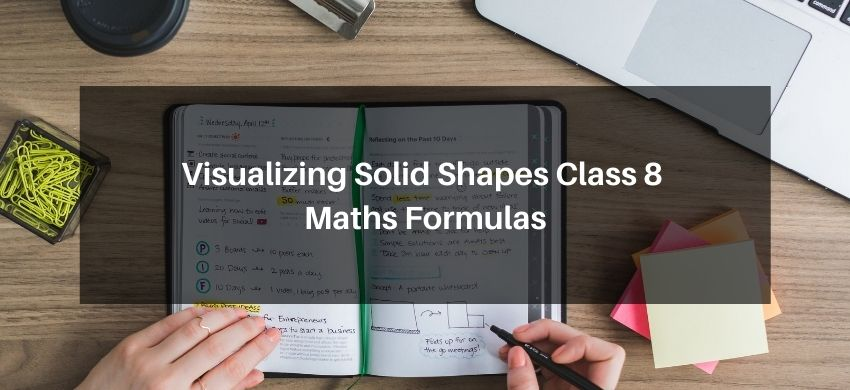# Visualizing Solid Shapes Class 8 Maths FormulasHey, students are you looking for Visualizing Solid Shapes Class 8 Maths Formulas? If yes. Then you are at the right place. In this post, I have listed all the formulas of Visualizing Solid Shape class 8 that you can use to learn and understand the concepts easily.

If you want to improve your class 8 Math, Visualizing Solid Shapes concepts, then it is super important for you to learn and understand all the formulas.

By using these formulas you will learn about the Visualizing Solid Shapes.

With the help of these formulas, you can revise the entire chapter easily.

## Visualizing Solid Shapes Class 8 Maths Formulas

• Prism: A prism is a solid, whose side faces are parallelograms and whose ends (or bases) are congruent parallel polygons. A prism has 2 triangular faces, 3 rectangular faces, 6 vertices and 9 edges.
• Pyramid: A pyramid is a polyhedron whose base is a polygon of any number of sides and whose other faces are triangles with a common vertex. A pyramid has 1 square face, 4 triangular faces, 5 vertices and 8 edges.
• Tetrahedron: A pyramid is called a triangular pyramid if its base is a triangle. A triangular pyramid is also called a tetrahedron.
• Plane shapes have two dimensions (measurements) like length and breadth whereas a solid object has three measurements like length, breadth and height (or depth). That is why they are called two-dimensional shapes and three-dimensional shapes, respectively. They are briefly named as 2-D and 3-D figures, respectively. Triangle, rectangle, circle, etc., are 2-D figures, whereas cube, cylinder, cone, sphere, etc., are 3-D figures.
• Views of 3-D Shapes: 3-dimensional objects look different from different positions. So, they can be drawn from different perspectives like top view, front view, side view.
• Mapping Space Around Us: A map is different from a picture. A map depicts the location of a particular object/place in relation to other objects/places. Symbols are used to depict different objects/places. There is no reference or perspective on a map. However, perspective is very important for drawing a picture. Moreover, maps involve a scale which is fixed for a particular map.
• Solids have a fixed shape and occupy a space.
• A solid is made up of polygonal regions, which are called its faces.
• Polyhedron: A solid shape bounded by polygons is called polyhedron (platonic solid).

A polyhedron has some number of plane faces, edges and vertices, which satisfy the relationship: F + V – E = 2,

F stands for the number of faces.

V stands for the number of vertices

and E stands for the number of edges.

The relation F + V – E = 2 is called Euler’s formula.

NCERT Class 8 Maths Book in PDF

NCERT Class 8 Science Book in PDF

NCERT Class 8 Science Exemplar in PDF

NCERT Class 8 Maths Exemplar in PDF

If you have any Confusion related to Visualizing Solid Shapes Class 8 Maths Formulas, then feel free to ask in the comments section down below.

To watch Free Learning Videos on Class 8 Maths by Kota’s top Faculties Install the eSaral App